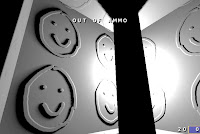## Wednesday, February 10, 2010

### Self-shadowing textures using radial horizon mapsI don't think I need to extoll the virtues of self-shadowing textures, so instead, I'll simply present a method for doing them:

Any given point on a heightmap can represent all of the shadowing from the surrounding heightmap as a waveform h = f(t), where t is a radial angle across the base plane, and h is the sine of the angle from the base plane to the highest-angled heightmap sample in that direction. h, in other words, is the horizon level for a light coming from that direction.

Anyway, the method for precomputing this should be pretty obvious: Fire traces from each sample and finding the shallowest angle that will clear all heightmap samples. Once you have it, it's simply a matter of encoding it, which can be easily done using Fourier series. Each Fourier band requires 2 coefficients, except the first which requires one since the sine band is zero. I use 5 coefficients (stored as an RGBA8 and A8), but 3 works acceptably. More coefficients requires more storage, but produces sharper shadows.

Fourier series are really straightforward, but here are the usual Cartesian coordinate spoilers:

Assuming x = sin(t) and y = cos(t)

[sin(0)] = 0
[cos(0)] = 1
[sin(n)] = x
[cos(n)] = y
[sin(2n)] = 2*x*y
[cos(2n)] = y*y - x*x

The constant band squared integrates to 1 over a circle, but all other bands integrate to 0.5. That means you need to double whatever you get from it to reproduce the original waveform.

There's one other problem, which is that the horizon will move rapidly at shallow angles, right where precision breaks down. You need more precision at low values, and my recommended way of doing that is storing the sign-preserving square root of the original value, and multiplying the stored value by the absolute value of itself in the shader.

For added effect, rather than simply doing a comparison of the light angle to the horizon level, you can take the difference of the two, multiply it by some constant, and saturate. This will produce a softer shadow edge.

Shader code to decode this, taking a texture coordinate and a tangent-space lighting direction to the light souce:

``float SelfShadowing(float3 tspaceLightDir, float2 tc){    float3 nLightDir = normalize(tspaceLightDir);    float4 lqCoefs = tex2D(pHorizonLQMap, tc) * 4.0 - 2.0;   // Premultiply by 2    float cCoef = tex2D(pHorizonCCUMap, tc).x * 2.0 - 1.0;    lqCoefs *= abs(lqCoefs);   // Undo dynamic range compression    cCoef *= abs(cCoef);    float2 tspaceRadial = normalize(float3(tspaceLightDir.xy, 0.0)).xy;    float3 qmultipliers = tspaceRadial.xyx * tspaceRadial.yyx * float3(2.0, 1.0, -1.0);    float horizonLevel = cCoef + dot(lqCoefs.rg, tspaceRadial)        + dot(lqCoefs.baa, qmultipliers);    return saturate(0.5 + 20.0*(tspaceLightDir.z - horizonLevel));}``

An additional optimization would be to exploit the fact that the range of the coefficients other than the constant band is not -1..1, but rather, -2/pi..2/pi. Expanding this to -1..1 gives you a good deal of additional precision.